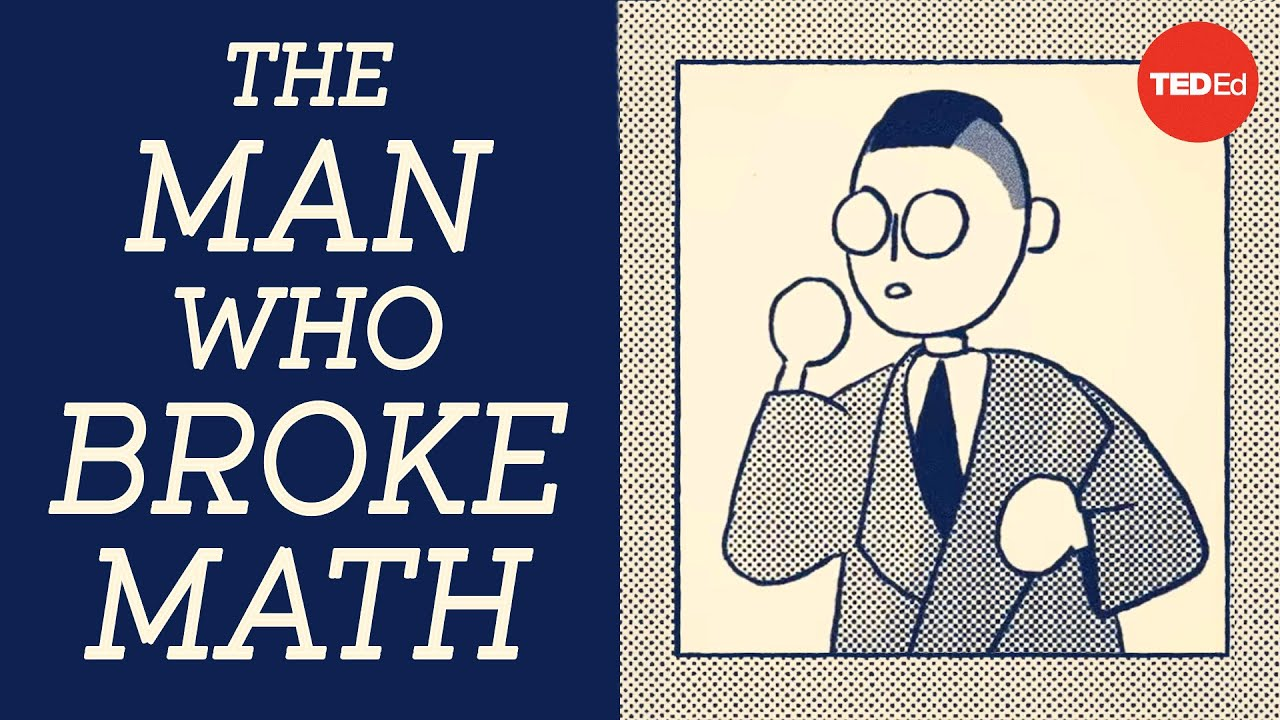Добавить новость# The paradox at the heart of mathematics: G del's Incompleteness Theorem - Marcus du Sautoy

Explore G del s Incompleteness Theorem, a discovery which changed what we know about mathematical proofs and statements.
Consider the following sentence: This statement is false. Is that true? If so, that would make the statement false. But if it s false, then the statement is true.
This…

Source: TED-Ed (2021-07-20)

Персональные новости